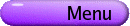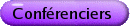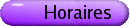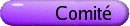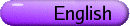Théorie des graphes
Org: Jing Huang, Kieka Mynhardt et Wendy Myrvold (Victoria)
[PDF]

RICHARD ANSTEE, UBC Mathematics, #121-1984 Mathematics Rd, Vancouver, BC
Robustness of the property of being matchable subject to vertex deletion
[PDF]

We consider classes of graphs which are easily seen to have many perfect matchings. The class of grid graphs is our main example. We then consider what properties to impose on choosing a subset of vertices A Í V(G) for vertex deletion in a graph G (from such a class) so that the vertex deleted subgraph G-A has a perfect matching. Certain conditions are easy. An even number of vertices must be deleted. If the graph is bipartite then the deleted vertices must have equal numbers from both parts of the bipartition. Also one cannot delete all the neighbours of a given vertex.

We obtain two results. In one, the deleted vertices are confined to the periphery of the graph and in the other the deleted vertices are required to be far apart. The motivation was a result of Jamison and Lockner presented at CGTC 34 (2003) but we can see this as an alternative to the edge extendibility investigations of Plummer et al.

ANTHONY BONATO, Wilfrid Laurier University, Waterloo, ON N2L 3C5
Random graph models and the web
[PDF]

Much recent attention has focussed on the rigorous design and analysis of models for the web graph and other massive self-organizing networks. Models for these networks often incorporate copying in their design, where a new node imperfectly duplicates some of the link structure of an existing node. The motivation for copying models comes from the fact that each node acts as an independent agent, which will base its decision on how to link to the existing network on local knowledge. As a result, the neighbourhood of a new node will often be similar to that of an existing node. Consideration of copying models and their limiting behaviour have led to the discovery of new connections between random graphs, graph homomorphisms, vertex pursuit games, and infinite graphs.

This is joint work with Manuel Bodirsky, Peter Cameron, Dejan Deli\'c, Jeannette Janssen, and Changping Wang.

RICK BREWSTER, Thompson Rivers University, Box 3010, Kamloops, BC V2C 3N5
Switch invariant homomorphism targets
[PDF]

A red-blue graph G is a set of vertices V(G) together with two edges sets: Er(G), the red edges; and Eb(G), the blue edges. Given red-blue graphs G and H, a homomorphism of G to H is a function f : V(G) ® V(H) such that uv Î Ei(G) implies f(u)f(v) Î Ei(H), for i Î {r,b}. We write G ®H.

Given a red-blue graph G and a vertex v Î V(G), the graph Gv is obtained from G by switching the colour of edge incident with v. (This process is analogous to Seidel switching when G is a complete red-blue graph.) A red-blue graph H is switch invariant if for any red-blue graph G we have G ® H if and only if Gv ® H for all v Î V(G). Switch invariant graphs arise in modeling certain constraint satisfaction problems.

We present a characterization of switch invariant red-blue graphs, plus generalize the problem to edge-coloured graphs with m edge colours.

This is joint work with Timothy Graves.

SEAN DAUGHERTY, University of Victoria
Exploring the Influence and Total Influence Numbers of a Graph
[PDF]

Recently, the study of social networks has given rise to a graph parameter known as the influence number: h(G). This parameter measures the influence that a vertex subset S has on the remaining vertices such that each vertex not in S is influenced by the closest member of S as follows: h(S) = åu Ï S2-d(u,S). The influence number of a graph is the maximum such value over all possible vertex subsets: h(G) = maxS Í Vh(S). A natural extension to the influence number allows each vertex not in S to be influenced by every vertex in S. This gives the total influence number: hT(G) = maxS Í V[åu Î S åv Ï S 2-d(u,v)]. Other applications include facility location problems where the quality of service decays exponentially with respect to distance. This talk will explore some of the basic results involving the influence and total influence numbers of a graph.

DANNY DYER, Memorial University of Newfoundland, St. John's, NL, A1C 5S7
Time constrained searching and sweeping
[PDF]

Graph searching and graph sweeping are often used as real world models in which mobile agents move to capture a mobile intruder. In graph searching, the intruder can only be located on vertices, while in graph sweeping, the intruder can also be located on edges. The most common problem examined with these models is to find the fewest agents which can guarantee the intruder's capture. However, in some situations, agents are "cheap", and we may then insist the intruder be captured quickly. We examine several such cases in variations of the the graph searching and sweeping models.

STEPHEN FINBOW, St. Francis Xavier University, PO Box 5000, Antigonish, Nova Scotia B2G 2W5
Generalisations of Domination, Independence and Irredundance
[PDF]

The study of the concepts of domination, independence and irredundance has led to many generalisations of these concepts in the literature. We consider a method for categorising many of these generalisations into a general framework. The framework is used to find connections between the generalised concepts and allows for many theorems (regarding these connections) to be proven at once.

SHANNON FITZPATRICK, University of Prince Edward Island
Independent Domination, Domination and Chromatic Number
[PDF]

An independent dominating set D is any set of vertices in a graph G such that no vertices in D are adjacent, but every vertex in V(G) - D is adjacent to a vertex in D. The independent domination number of G, denoted i(G), is the cardinality of a minimum independent dominating set.

The following upper bounds have been found by Bollobás & Cockayne and MacGillivray & Seyffarth, respectively:

 i(G) £ n - g(G) - éê n-g(G) g(G) ùú + 1
 i(G) £ n - c(G) - éê n-c(G) c(G) ùú + 1
where n=|V(G)|, g(G) is the domination number of G and c(G) is the chromatic number of G. I will discuss the characterization of those graphs for which equality is achieved for the first upper bound and the problem of determining those instances for which the first upper bound provides a better result than the second.

PATRICK FOWLER, University of Sheffield, UK
Cospectrality and the fullerenes
[PDF]

A fullerene polyhedron, representing an all-carbon molecule Cn, is cubic, with only pentagonal and hexagonal faces (12 and n/2-10, respectively, by Euler's theorem). The spectrum of the adjacency matrix contains a great deal of qualitative chemical information on optimum electron count, electronic configuration, stability and reactivity of the molecule. Connections between graph theory and these chemical models will be discussed. Cospectral fullerene graphs would be of interest as representing molecules with structures that were different but with (some) identical properties. As yet, no cospectral fullerenes are known, but many pairs of fullerenes with cospectral duals have now been found (P. W. Fowler and M. Duncan, to be published). The dual of a fullerene graph is also a polyhedral graph, having all faces triangular, 12 vertices of degree 5 and all others of degree 6; considered as the skeleton of a molecule, it is a model for large boron hydrides, based on extrapolation of the known closo-series of borane anions, BN HN2-. A construction for (infinite) families of cospectral fullerene duals will be presented.

LUIS GODDYN, Simon Fraser University, Burnaby, BC V5A 1S6
Silver Cubes
[PDF]

An n ×n matrix is silver if, for i=1,...,n, every symbol in {1,2,...,2n-1} appears as an entry in either row i or column i.

An IMO 1997 question introduced this definition, and asked whether a silver matrix of order 1997 exists. (In fact, one exists if and only if n=1 or n is even.) In this paper we investigate higher dimensional analogs, silver cubes.

Finding the correct generalization is the first challenge. The cells on the main diagonal of a silver matrix are treated specially. What should serve as a "diagonal" in a d-dimensional n×n×¼×n silver cube? We propose that a "diagonal" should be a "maximum independent set in the d-th cartesian power of the complete graph of order n." This definition is motivated by "minimal defining sets" for colouring such graphs. The challenge is to label the cells with symbols 1,2,...,d(n-1)+1 so that, for each cell c on the "diagonal", every symbol appears once in one of the d(n-1)+1 cells orthogonally translated from c. We present constructions, nonexistence proofs and connections with coding theory and projective geometry.

This is joint work with M. Ghebleh, E. Mahmoodian and M. Verdian-Rizi.

GENA HAHN, Université de Montréal
Recent bounds on the injective chromatic number
[PDF]

We introduced the injective chromatic number in  but it had been studied in different guises before. In this talk we review previous results and give new bounds on the number for some classes of graphs.

This is joint work with Alain Doyon, André Raspaud and Weifan Wang.

## References


G. Hahn, J. Kratochvíl, D. Sotteau and J. Sirán, On injective colourings. Discrete Math. 256(2002), 179-192.

BERT HARTNELL, Saint Mary's University, Halifax, Nova Scotia B3H 3C3
Monitoring a Network with fewer Watchpeople: the trade offs
[PDF]

A dominating set S of a graph is a set of vertices such that every vertex of the graph is either in S or adjacent to some vertex of S. In particular, the dominating set is considered static and all vertices of the graph are dominated all of the time. In many applications this may not be practical as resources are simply too limited. Hence we might allow vertices to not be dominated for certain periods of time as long as such time intervals are not excessive. Thus we could allow each member of our set to either remain fixed or move to an adjacent vertex in one unit of time. What can be said about the time vertices are not monitored if the dominating set is cut to some fraction of the original number required or by a fixed number? Although more questions than answers will be given, some observations will be outlined.

NORA HARTSFIELD, Western Washington University, Bellingham, WA 98225-9063, USA
Self-dual embeddings of strongly regular self-complementary graphs
[PDF]

An infinite family of self-dual embeddings of strongly regular self-complementary graphs is presented. These embeddings are very highly structured and have the property that if one draws the dual on the same surface, without moving any vertices, the resulting embedding of the complement is also self-dual and all four embeddings are map isomorphic.

PETER HORAK, University of Washington, Tacoma
Perfect dominating sets
[PDF]

A perfect dominating set S of distance d in a graph G is a set of its vertices so that each vertex of G is at distance at most d from exactly one vertex of S. In 1968 Golomb and Welsh conjectured (they formulated their conjecture in terms of Lee-distance error correcting codes) that a perfect dominating set of distance d in the n-dimensional torus Ck ×¼×Ck exists only in the case for n=1 and any d or n=2 and any d or d=1 and any n. Despite a lot of effort by researchers both in graph theory and in coding theory the conjecture is still open although there are plenty of partial results supporting the conjecture. In this talk we will discuss some variations of the conjecture that are motivated by an application in computer science.

ROSS KANG, Oxford University, Department of Statistics, 1 South Parks Road, Oxford OX1 3TG, United Kingdom
The t-improper chromatic number of random graphs
[PDF]

We consider the t-dependence and t-improper chromatic numbers of the Erdös-Rényi random graph. As usual, Gn,p denotes a random graph with vertex set [n] = {1,...,n} in which the edges are chosen independently at random with probability p. The t-dependence number at(G) of a graph G is the maximum size of a t-dependent set-a vertex subset which induces a subgraph of maximum degree at most t. The t-improper chromatic number ct(G) is the smallest number of colours needed in a t-improper colouring-a colouring of the vertices in which colour classes are t-dependent sets. Note that ct(G) ³ |V(G)|/at(G) and ct(G) ³ ct+1(G) for any graph G.

Clearly, when t = 0, we are considering the independence number and chromatic number of random graphs, and the problem of determining the asymptotic behaviour of the chromatic number c(Gn,p) was once one of the central open problems in random graph theory. We consider a fixed edge-probability p where 0 < p < 1, and t=t(n). Our results on ct(Gn,p) break into three ranges for t(n). We say that a property holds asymptotically almost surely (a.a.s.) if it holds with probability ® 1 as n ® ¥.

(a) If t(n) = o(logn), then ct(Gn,p) ~ ([ 1/2]log[ 1/(1-p)]) [(n)/(logn)] a.a.s. Thus, if the impropriety t does not grow too fast, then the t-improper chromatic number is likely to be close to the proper chromatic number.
(b) If t(n) = Q(logn), then ct(Gn,p) = Q([(np)/(t)]) a.a.s.
(c) Lastly, if t(n)/logn ® ¥, then ct(Gn,p) ~ [(np)/(t)] a.a.s.

This is joint work with Colin McDiarmid.

BILL KOCAY, University of Manitoba
Degree Sequence Problems for 3-Hypergraphs
[PDF]

The Erdös-Gallai conditions are necessary and sufficient conditions for the existence of a simple graph with a given degree sequence. Much work has been done characterizing the polytope of degree sequences of simple graphs. The corresponding conditions for 3-hypergraphs are still unknown.

A simple 3-hypergraph G consists of a set V of vertices and E of edges, such that each edge is a triple u,v,w of distinct vertices. Repeated triples are not allowed in G. The degree of a vertex v is deg(v), the number of triples containing v. The degree sequence of G is the sequence of degrees D(G) = (d1,d2,...,dn), such that d1 ³ d2 ³ ¼ ³ dn. We ask when a given sequence D is the degree sequence of a simple 3-hypergraph?

It is still unknown whether this problem has a polynomial-time algorithmic solution, or whether it is NP-complete. Recently Kocay and Li showed that any two 3-hypergraphs with the same degree sequence can be transformed into each other by a sequence of operations known as trades. The proof is based on null-hypergraphs. We describe the structure of null-hypergraphs, and a closely related NP-complete problem for 3-hypergraph degree sequences.

JIM LIU, University of Lethbridge
On the recognition of probe graphs of some self-complementary classes of perfect graphs
[PDF]

In this paper we consider the recognition of some probe graph classes. Given a class of graphs G, a graph G is a probe graph of G if its vertices can be partitioned into a set P of probes and an independent set N of nonprobes, such that G can be extended to a graph of G by adding edges between certain nonprobes. We show that there are polynomial-time recognition algorithms for probe cographs, probe P4-reducible graphs, probe P4-sparse graphs, and probe splitgraphs.

Joint work with Maw-Shang Chang, Ton Kloks, Dieter Kratsch and Sheng-Lung Peng.

JOY MORRIS, University of Lethbridge, Lethbridge, AB T1K 6R4
Cyclic Hamiltonian (Near-)Decompositions of the Complete Graph
[PDF]

It has been proven that the complete graph on n vertices can be decomposed into Hamiltonian cycles, whenever n is odd. Similarly, if we remove a 1-factor (perfect matching) from the complete graph on an even number of vertices, the remaining graph can always be decomposed into Hamiltonian cycles; this is what is referred to as a near-decomposition. To make the problem interesting again, we put constraints on the Hamiltonian cycles that we allow to be in the decomposition. A cyclic Hamiltonian decomposition of the complete graph is a decomposition of the complete graph into Hamiltonian cycles, in such a way as to ensure that rotating any cycle in the decomposition gives us a (possibly different) cycle in the decomposition. It has been proven that a cyclic Hamiltonian decomposition of the complete graph on n vertices always exists when n is odd, as long as n ¹ 15 and n ¹ pa, where p is prime and a > 1, and that these constraints are necessary. We prove that when n is even, cyclic Hamiltonian near-decompositions of the complete graph on n vertices exist if and only if n ¹ 2pa where p is prime, and n is either 2 or 4 (mod 8).

WENDY MYRVOLD, University of Victoria, Dept. of Computer Science
Fast Enumeration of All Independent Sets of a Graph
[PDF]

An independent set of a graph G is a set of vertices of G which are pairwise non-adjacent. There are many applications for which the input is a graph G with a large symmetry group and the goal is to generate either all of the independent sets or all of the maximum independent sets up to isomorphism. We present a very fast practical algorithm for this problem. The tactic can also be applied to many other problems: some examples are generation of all colourings or matchings of a graph up to isomorphism. Two applications are given to illustrate the utility of this algorithm: finding all independent sets of the C60 and C70 fullerenes, and also the problem of finding a maximum set of vertices at distance four or more in the 120-cell.

This is joint work with Patrick Fowler.

ORTRUD OELLERMANN, University of Winnipeg, 515 Portage Avenue, Winnipeg
The Strong Metric Dimension of a Graph
[PDF]

Let G be a connected graph. A vertex w is said to strongly resolve a pair u,v of vertices of G if there exists some shortest u-w path containing v or some shortest v-w path containing u. A set W of vertices is a strong resolving set for G if every pair of vertices of G is strongly resolved by some vertex of W. A smallest strong resolving set for G is called a strong basis for G and its cardinality the strong dimension of G. We begin with a motivation and an overview of this invariant and a related invariant, namely the metric dimension of a graph. We then show that the problem of finding the strong dimension of a connected graph can be transformed to the problem of finding the vertex covering number of a graph. Moreover, this invariant is shown to be NP-hard.

Joint work with J. Peters-Fransen.

DAVID PIKE, Memorial University of Newfoundland, St. John's, Newfoundland
Pancyclic PBD block-intersection graphs
[PDF]

A pairwise balanced design PBD(v,K,l) consists of a set V of cardinality v, a set K of positive integers, and a set B of subsets of V with the properties that |b| Î K for each b Î B, and each pair of elements from V occurs in exactly l of the subsets in B. The elements of B are known as the blocks of the design.

Given a combinatorial design D with block set B, its block-intersection graph GD is the graph having vertex set B such that two vertices b1 and b2 are adjacent if and only if b1 and b2 have non-empty intersection.

Hare showed in 1995 that if D is a PBD(v,K,1) with minK \geqslant 3, then GD is edge-pancyclic (i.e., each edge of GD is contained in a cycle of each length l = 3,4,...,|V(GD)|). In this presentation we consider block-intersection graphs of pairwise balanced designs PBD(v,K,l) for which l\geqslant 2.

This is joint work with Graham Case.

MATEJA SAJNA, University of Ottawa, Department of Mathematics and Statistics, 585 King Edward Avenue, Ottawa, ON K1N 6N5
Two models for transitive closure of bipolar weighted digraphs
[PDF]

A bipolar weighted digraph is a digraph together with a weight function and a sign function on the arcs such that the weight of each arc lies in the interval [0,1] and no two parallel arcs have the same sign. Bipolar weighted digraphs are natural models for so-called fuzzy cognitive maps, which are used in science, engineering, and the social sciences to represent a body of knowledge. It has been noted in the literature that a transitive closure of a bipolar weighted digraph contains useful new information for the fuzzy cognitive map it models.

It is natural to define a transitive closure of a bipolar weighted digraph D=(V,A) as a bipolar weighted digraph D*=(V,A*) such that an arc (u,v) of sign s is in A* if and only if D has a directed (u,v)-walk of sign s (where the sign of a directed walk is defined as the product of signs of all its arcs). But what weight should be assigned to (u,v) in D*? We propose two models: the bipolar fuzzy digraph model, which has been previously considered in some form in fuzzy systems research, and the new bipolar random digraph model. A bipolar fuzzy digraph consists of two fuzzy relations on the set V (that is, the arc weights are viewed as degrees of membership), and its transitive closure is a combined transitive closure of the two fuzzy relations. In a random digraph model, on the other hand, the arc weights are viewed as probabilities, and the weight of an arc (u,v) of sign s in the transitive closure is defined as the probability of having a directed (u,v)-walk of sign s in D. While a version of Roy-Warshall's algorithm efficiently computes the transitive closure of a bipolar fuzzy digraph, the problem is computationally hard for bipolar random digraphs. However, we describe several approaches that allow computation at least for the types and sizes of fuzzy cognitive maps we have dealt with in practice.

JOZSEF SOLYMOSI, University of British Columbia, Vancouver
Extremal problems for linear k-uniform hypergraphs
[PDF]

A hypergraph is linear if every two hyperedges share one point at most. We investigate the following question. What is the maximum possible number of edges in a linear k-uniform hypergraph H with n vertices which does not contain a given linear k-uniform hypergraph G as a subgraph? We show applications to geometry and number theory.

LADISLAV STACHO, Simon Fraser University, Burnaby
Ordered k-colorings dichotomy
[PDF]

We introduce three variants of proper colorings with imposed partial ordering on the set of colors and will present dichotomy theorems that separate these problems into tractable and NP-complete.

Vertices of all considered graphs are integers from 1 to |V(G)|, hence they form a linearly ordered set (V (G), £ ). The set of vertices colored c will be denoted by Vc. Given a partially ordered set (poset) (C,\preceq) of colors, in the first problem we want to (properly) color vertices of G by colors in C (color G by poset C) such that for any two colors c and c¢ if c\preceq c¢ then for any two vertices u Î Vc and v Î Vc¢, u £ v. Thus, if \preceq is the empty relation on C, then the problem is whether G can be properly colored with |C| colors, a well known graph coloring problem.

In the second problem, we want to color G by poset C such that for any two colors c and c¢ if c\preceq c¢ then for any two adjacent vertices u Î Vc and v Î Vc¢, u £ v. This problem is the well-known directed graph homomorphism problem whose dichotomy was extensively studied.

In the last problem, we want to color G by poset C such that for any two colors c and c¢ if c\preceq c¢ then for any two vertices u Î Vc and v Î Vc¢ in a component induced by VcÈVc¢, u £ v.

This is a joint work with Arvind Gupta, Jan van den Heuvel, Jan Manuch, and Xiaohong Zhao.

BAOGANG XU, Nanjing Normal University, Nanjing, 210097, P.R. China
Some results on minimally circular-imperfect graphs
[PDF]

A graph is circular-perfect if each of its induced subgraphs has the same circular chromatic number as its circular clique number. A graph is called minimally circular-imperfect if the graph itself is not circular-perfect but each of its proper induced subgraphs is. One approach to study the circular-perfect graphs is to characterize the minimally circular-imperfect graphs. In this talk, some results on minimally circular-imperfect graphs are presented.